# Count Subsets with Sum K (DP – 17)

Problem Statement: Count Subsets with Sum K

Problem Link: Count Subsets With Sum K

We are given an array ‘ARR’ with N positive integers and an integer K. We need to find the number of subsets whose sum is equal to K.

```Example: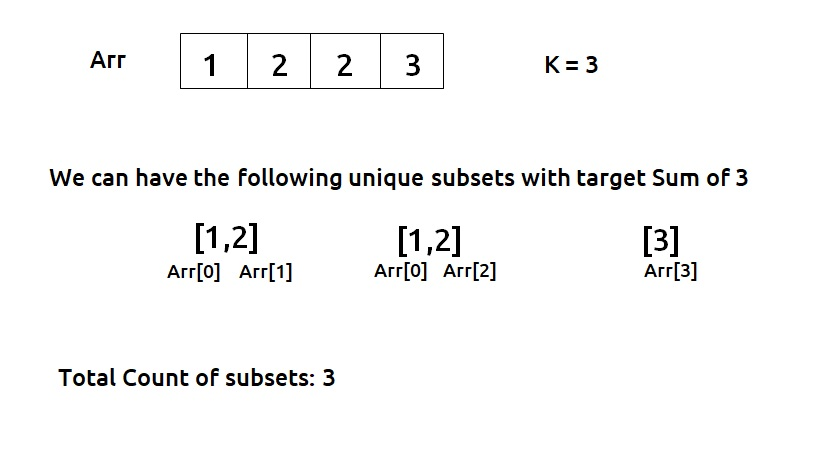```

Disclaimer: Don’t jump directly to the solution, try it out yourself first.

### Solution :

A Greedy Solution doesn’t make sense because we are not looking to optimize anything. We can rather try to generate all subsequences using recursion and whenever we get a single subsequence whose sum is equal to the given target, we can count it.

Note: Readers are highly advised to watch this video “Recursion on Subsequences” to understand how we generate subsequences using recursion.

### Steps to form the recursive solution:

We will first form the recursive solution by the three points mentioned in Dynamic Programming Introduction

Step 1: Express the problem in terms of indexes.

The array will have an index but there is one more parameter “target”. We are given the initial problem to find whether there exists in the whole array a subsequence whose sum is equal to the target.

So, we can say that initially, we need to find(n-1, target) which means that we are counting the number of subsequences in the array from index 0 to n-1, whose sum is equal to the target. Similarly, we can generalize it for any index ind as follows: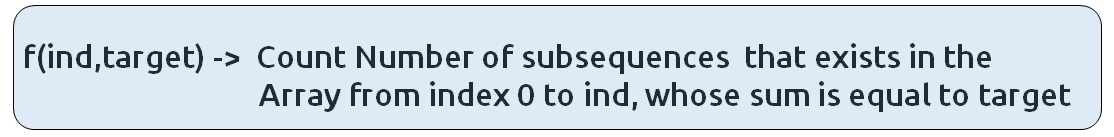### Base Cases:

• If target == 0, it means that we have already found the subsequence from the previous steps, so we can return 1.
• If ind==0, it means we are at the first element, so we need to return arr[ind]==target. If the element is equal to the target we return 1 else we return 0.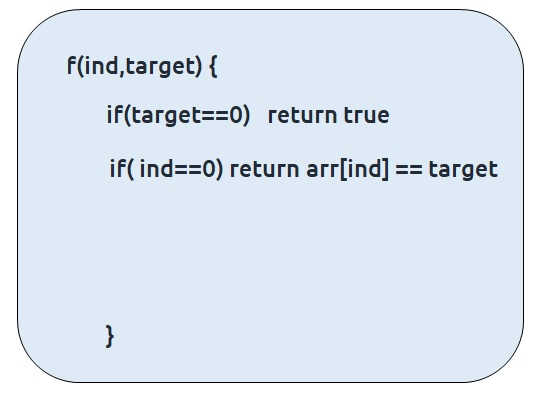Step 2: Try out all possible choices at a given index.

We need to generate all the subsequences. We will use the pick/non-pick technique as discussed in this video “Recursion on Subsequences”.

We have two choices:

• Exclude the current element in the subsequence: We first try to find a subsequence without considering the current index element. For this, we will make a recursive call to f(ind-1,target).
• Include the current element in the subsequence: We will try to find a subsequence by considering the current index as element as part of subsequence. As we have included arr[ind], the updated target which we need to find in the rest if the array will be target – arr[ind]. Therefore, we will call f(ind-1,target-arr[ind]).

Note: We will consider the current element in the subsequence only when the current element is less than or equal to the target.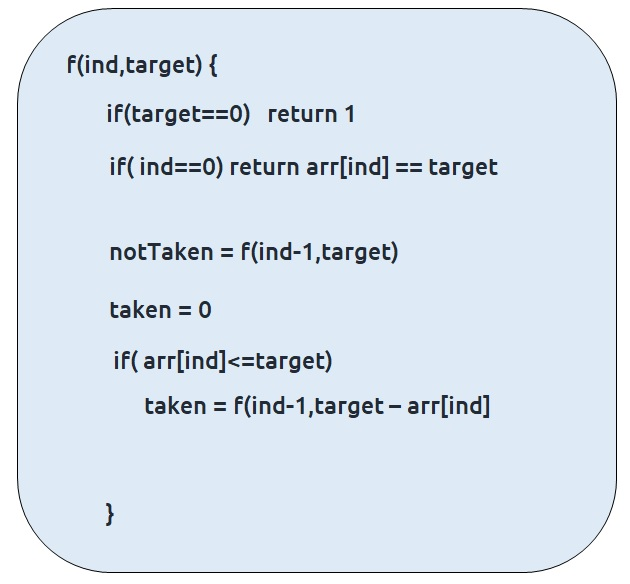Step 3:  Return sum of taken and notTaken

As we have to return the total count of subsets with the target sum, we will return the sum of taken and notTaken from our recursive call.

The final pseudocode after steps 1, 2, and 3:Steps to memoize a recursive solution:

If we draw the recursion tree, we will see that there are overlapping subproblems. In order to convert a recursive solution the following steps will be taken:

1. Create a dp array of size [n][k+1]. The size of the input array is ‘n’, so the index will always lie between ‘0’ and ‘n-1’. The target can take any value between ‘0’ and ‘k’. Therefore we take the dp array as dp[n][k+1]
2. We initialize the dp array to -1.
3. Whenever we want to find the answer of particular parameters (say f(ind,target)), we first check whether the answer is already calculated using the dp array(i.e dp[ind][target]!= -1 ). If yes, simply return the value from the dp array.
4. If not, then we are finding the answer for the given value for the first time, we will use the recursive relation as usual but before returning from the function, we will set dp[ind][target] to the solution we get.

Code:

## C++ Code

``````#include <bits/stdc++.h>

using namespace std;

int findWaysUtil(int ind, int target, vector<int>& arr, vector<vector<int>> &dp){
if(target==0)
return 1;

if(ind == 0)
return arr == target;

if(dp[ind][target]!=-1)
return dp[ind][target];

int notTaken = findWaysUtil(ind-1,target,arr,dp);

int taken = 0;
if(arr[ind]<=target)
taken = findWaysUtil(ind-1,target-arr[ind],arr,dp);

return dp[ind][target]= notTaken + taken;
}

int findWays(vector<int> &num, int k){
int n = num.size();
vector<vector<int>> dp(n,vector<int>(k+1,-1));
return findWaysUtil(n-1,k,num,dp);
}

int main() {

vector<int> arr = {1,2,2,3};
int k=3;

cout<<"The number of subsets found are " <<findWays(arr,k);
}
``````

Output:

The number of subsets found are 3

Time Complexity: O(N*K)

Reason: There are N*K states therefore at max ‘N*K’ new problems will be solved.

Space Complexity: O(N*K) + O(N)

Reason: We are using a recursion stack space(O(N)) and a 2D array ( O(N*K)).

## Java Code

``````import java.util.*;

class TUF{
static int findWaysUtil(int ind, int target, int[] arr,int[][] dp){
if(target==0)
return 1;

if(ind == 0)
return arr == target?1:0;

if(dp[ind][target]!=-1)
return dp[ind][target];

int notTaken = findWaysUtil(ind-1,target,arr,dp);

int taken = 0;
if(arr[ind]<=target)
taken = findWaysUtil(ind-1,target-arr[ind],arr,dp);

return dp[ind][target]= notTaken + taken;
}

static int findWays(int[] num, int k){
int n = num.length;
int dp[][]=new int[n][k+1];
for(int row[]: dp)
Arrays.fill(row,-1);

return findWaysUtil(n-1,k,num,dp);
}

public static void main(String args[]) {

int arr[] = {1,2,2,3};
int k=3;

System.out.println("The number of subsets found are "+findWays(arr,k));
}
}``````

Output:

The number of subsets found are 3

Time Complexity: O(N*K)

Reason: There are N*K states therefore at max ‘N*K’ new problems will be solved.

Space Complexity: O(N*K) + O(N)

Reason: We are using a recursion stack space(O(N)) and a 2D array ( O(N*K)).

Steps to convert Recursive Solution to Tabulation one.

To convert the memoization approach to a tabulation one, create a dp array with the same size as done in memoization. We can initialize it as 0.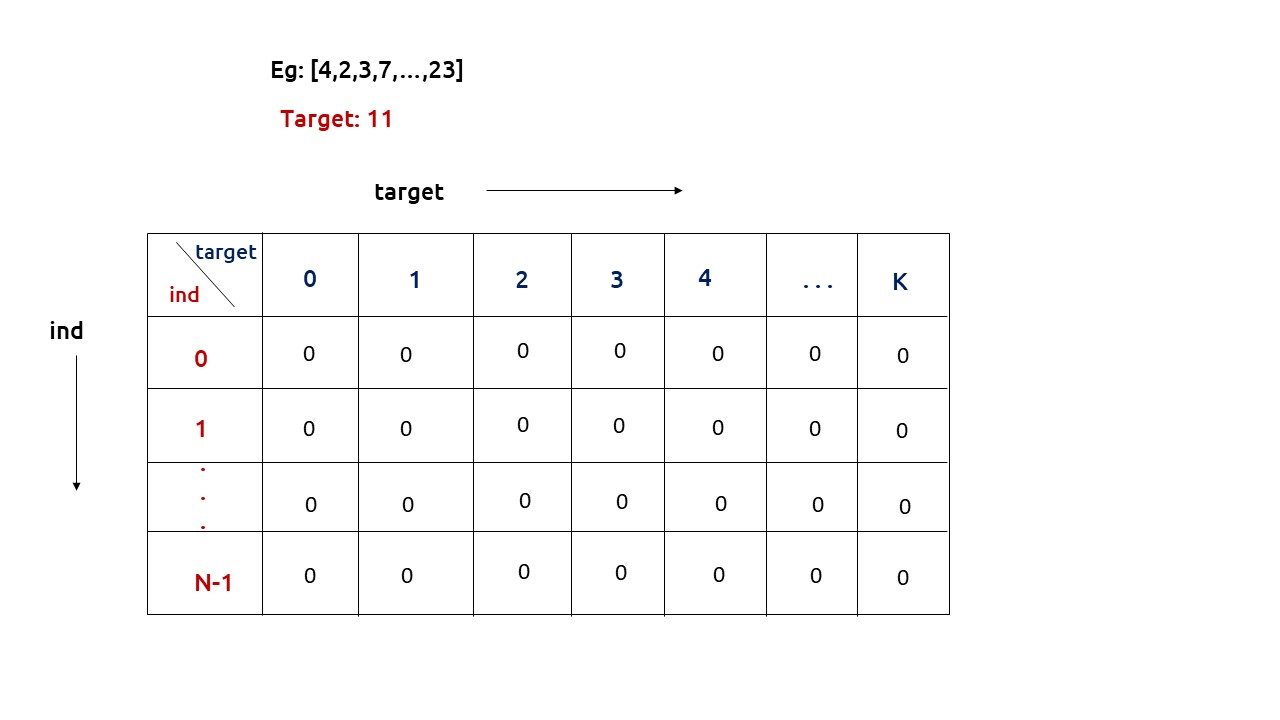First, we need to initialize the base conditions of the recursive solution.

• If target == 0, ind can take any value from 0 to n-1, therefore we need to set the value of the first column as 1.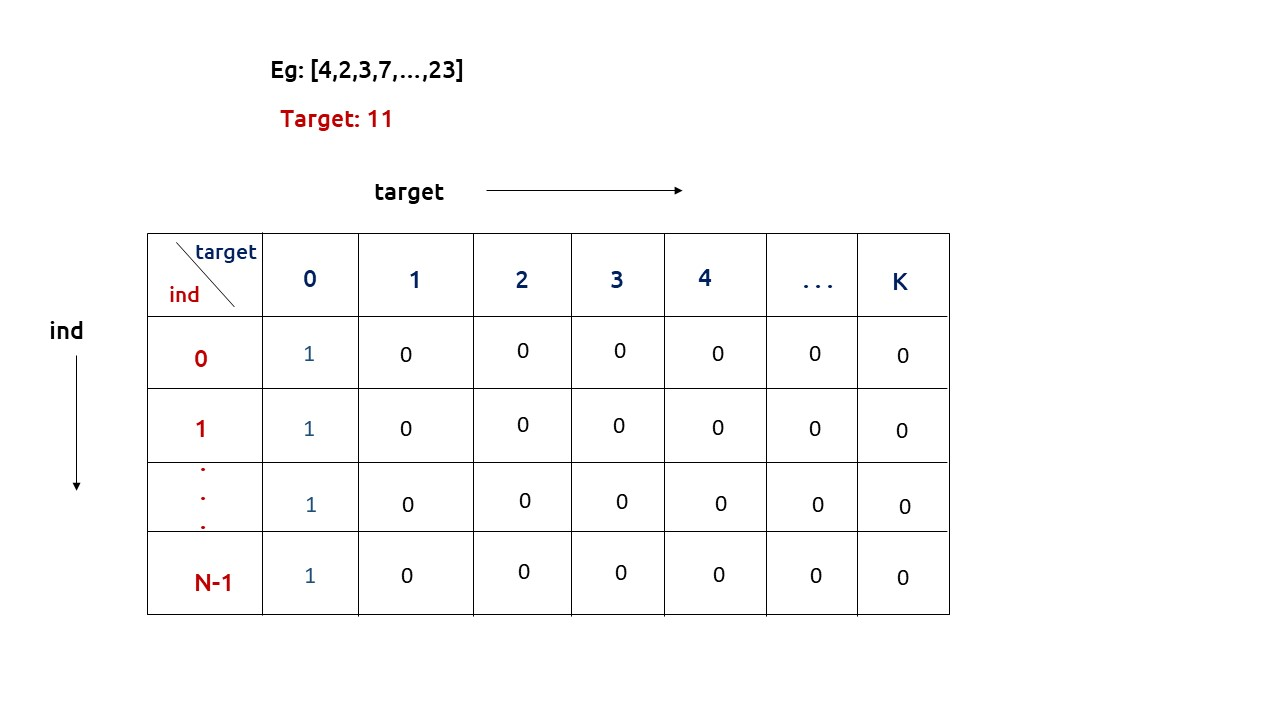• The first row dp[] indicates that only the first element of the array is considered, therefore for the target value equal to arr, only cell with that target will be true, so explicitly set dp[arr] =1, (dp[arr] means that we are considering the first element of the array with the target equal to the first element itself). Please note that it can happen that arr>target, so we first check it: if(arr<=target) then set dp[arr] = 1.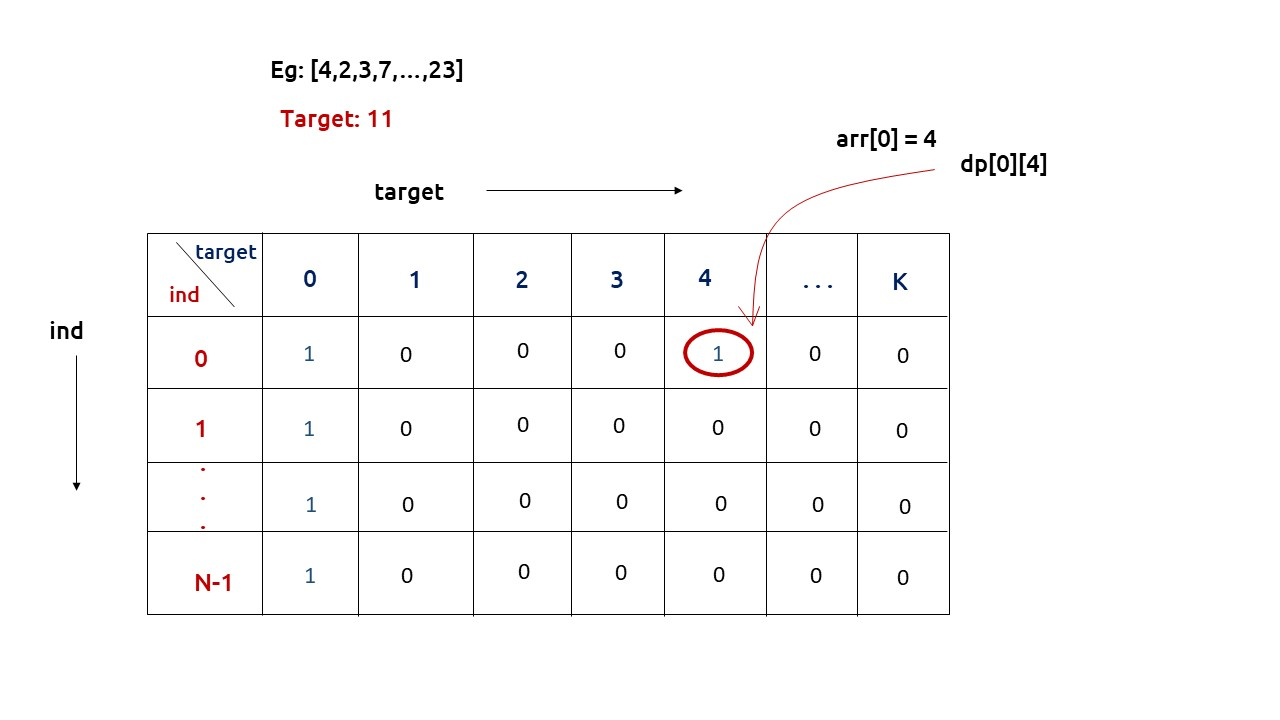• After that , we will set our nested for loops to traverse the dp array and following the logic discussed in the recursive approach, we will set the value of each cell. Instead of recursive calls, we will use the dp array itself.
• At last we will return dp[n-1][k] as our answer.

Code:

## C++ Code

``````#include <bits/stdc++.h>

using namespace std;

int findWays(vector<int> &num, int k){
int n = num.size();

vector<vector<int>> dp(n,vector<int>(k+1,0));

for(int i=0; i<n; i++){
dp[i] = 1;
}

if(num<=k)
dp[num] = 1;

for(int ind = 1; ind<n; ind++){
for(int target= 1; target<=k; target++){

int notTaken = dp[ind-1][target];

int taken = 0;
if(num[ind]<=target)
taken = dp[ind-1][target-num[ind]];

dp[ind][target]= notTaken + taken;
}
}

return dp[n-1][k];

}

int main() {

vector<int> arr = {1,2,2,3};
int k=3;

cout<<"The number of subsets found are " <<findWays(arr,k);
}
``````

Output:

The number of subsets found are 3

Time Complexity: O(N*K)

Reason: There are two nested loops

Space Complexity: O(N*K)

Reason: We are using an external array of size ‘N*K’. Stack Space is eliminated.

## Java Code

``````import java.util.*;

class TUF{

static int findWays(int[] num, int k){
int n = num.length;

int[][] dp=new int[n][k+1];

for(int i=0; i<n; i++){
dp[i] = 1;
}

if(num<=k)
dp[num] = 1;

for(int ind = 1; ind<n; ind++){
for(int target= 1; target<=k; target++){

int notTaken = dp[ind-1][target];

int taken = 0;
if(num[ind]<=target)
taken = dp[ind-1][target-num[ind]];

dp[ind][target]= notTaken + taken;
}
}

return dp[n-1][k];

}

public static void main(String args[]) {

int arr[] = {1,2,2,3};
int k=3;

System.out.println("The number of subsets found are "+findWays(arr,k));
}
}``````

Output:

The number of subsets found are 3

Time Complexity: O(N*K)

Reason: There are two nested loops

Space Complexity: O(N*K)

Reason: We are using an external array of size ‘N*K’. Stack Space is eliminated.

Part 3: Space Optimization

If we closely look the relation,

dp[ind][target] =  dp[ind-1][target] + dp[ind-1][target-arr[ind]]

We see that to calculate a value of a cell of the dp array, we need only the previous row values (say prev). So, we don’t need to store an entire array. Hence we can space optimize it.

Note: Whenever we create a new row ( say cur), we need to explicitly set its first element is true according to our base condition.

Code:

## C++ Code

``````#include <bits/stdc++.h>

using namespace std;

int findWays(vector<int> &num, int k){
int n = num.size();

vector<int> prev(k+1,0);

prev =1;

if(num<=k)
prev[num] = 1;

for(int ind = 1; ind<n; ind++){
vector<int> cur(k+1,0);
cur=1;
for(int target= 1; target<=k; target++){

int notTaken = prev[target];

int taken = 0;
if(num[ind]<=target)
taken = prev[target-num[ind]];

cur[target]= notTaken + taken;
}

prev = cur;
}

return prev[k];

}

int main() {

vector<int> arr = {1,2,2,3};
int k=3;

cout<<"The number of subsets found are " <<findWays(arr,k);
}
``````

Output:

The number of subsets found are 3

Time Complexity: O(N*K)

Reason: There are three nested loops

Space Complexity: O(K)

Reason: We are using an external array of size ‘K+1’ to store only one row.

## Java Code

``````import java.util.*;

class TUF{

static int findWays(int[] num, int k){
int n = num.length;

int prev[]=new int[k+1];

prev =1;

if(num<=k)
prev[num] = 1;

for(int ind = 1; ind<n; ind++){
int cur[]=new int[k+1];
cur=1;
for(int target= 1; target<=k; target++){

int notTaken = prev[target];

int taken = 0;
if(num[ind]<=target)
taken = prev[target-num[ind]];

cur[target]= notTaken + taken;
}

prev = cur;
}

return prev[k];

}

public static void main(String args[]) {

int arr[] = {1,2,2,3};
int k=3;

System.out.println("The number of subsets found are "+findWays(arr,k));
}
}``````

Output:

The number of subsets found are 3

Time Complexity: O(N*K)

Reason: There are two nested loops

Space Complexity: O(K)

Reason: We are using an external array of size ‘K+1’ to store only one row.

Special thanks to Anshuman Sharma for contributing to this article on takeUforward. If you also wish to share your knowledge with the takeUforward fam, please check out this article